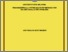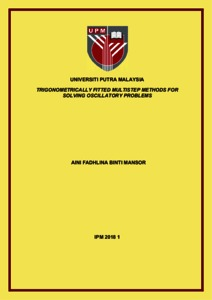# Trigonometrically fitted multistep methods for solving oscillatory problems

## Citation

Mansor, Aini Fadhlina (2017) Trigonometrically fitted multistep methods for solving oscillatory problems. Masters thesis, Universiti Putra Malaysia.

## Abstract

At the beginning of the thesis, we trigonometrically fitted the first point of the existing block multistep method which was purposely derived for solving special second order ordinary differential equations (ODEs). Based on the original multistep method, we construct both explicit and implicit trigonometrically fitted multistep methods of step number k=4 and develop code using both constant and variable step size. The trigonometrically fitting technique has been applied to the original method in order to construct the new methods. The numerical results show that the trigonometrically fitted multistep method is more efficient compared to the existing methods in solving special second order ordinary differential equations (ODEs) which are oscillatory in nature. Then, the 2-point explicit and implicit block multistep methods of step number k=3 and k=5 for solving special second order ODEs are derived using integration formula based on Newton-Gregory backward interpolation polynomial. The methods are implemented for constant step size by using the predictor-corrector technique, followed by the implementation using variable step size. The numerical results are given to show the efficiency of the new methods as compared to the existing methods. The 2-point explicit and implicit block multistep methods of step number k=3 and k=5 are then trigonometrically fitted so that they are suitable for solving special second order ordinary differential equations, which are highly oscillatory in nature. We developed codes based on the trigonometrically fitted methods using constant step size in predictor-corrector mode. The numerical results obtained show that trigonometrically fitted the methods give more accurate solutions than the existing methods. In conclusion, trigonometrically fitted block and non-block multistep methods have been derived in this thesis for solving oscillatory problems. The illustrative examples are given in the form of both tables and graphs which clearly shown the advantage of the methods.Preview
Text
IPM 2018 1 IR.pdfView Item